﻿ 五分钟搞定删除excel单元格空格的问题 - 自由资讯

# 五分钟搞定删除excel单元格空格的问题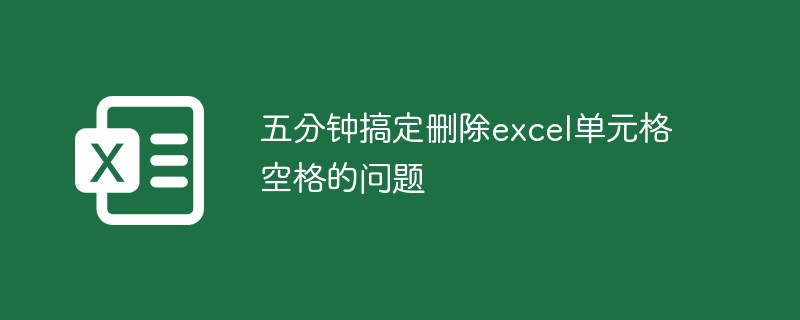## 1、 正经的空格删除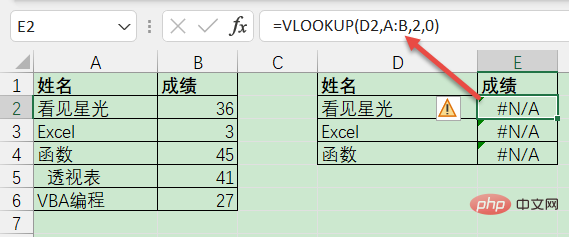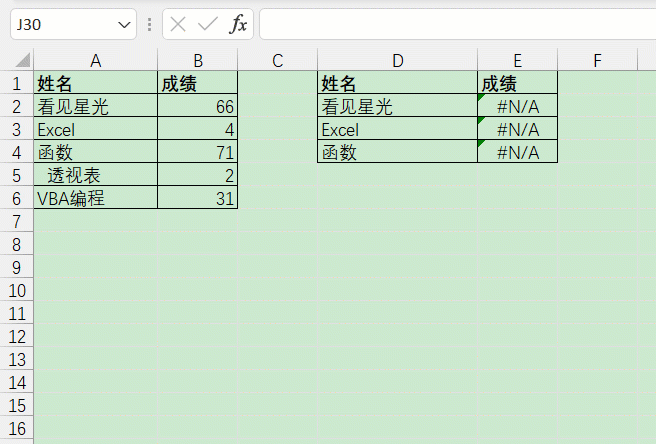## 2、身份证中的空格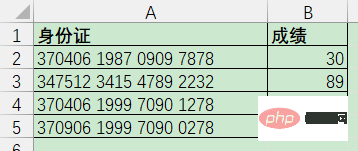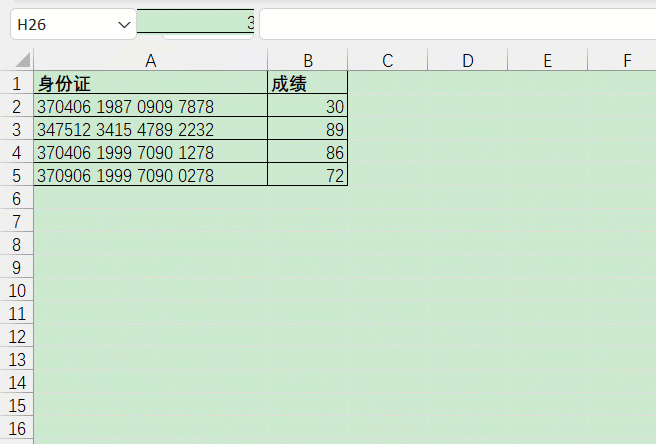`=SUBSTITUTE(A2,” “,””)`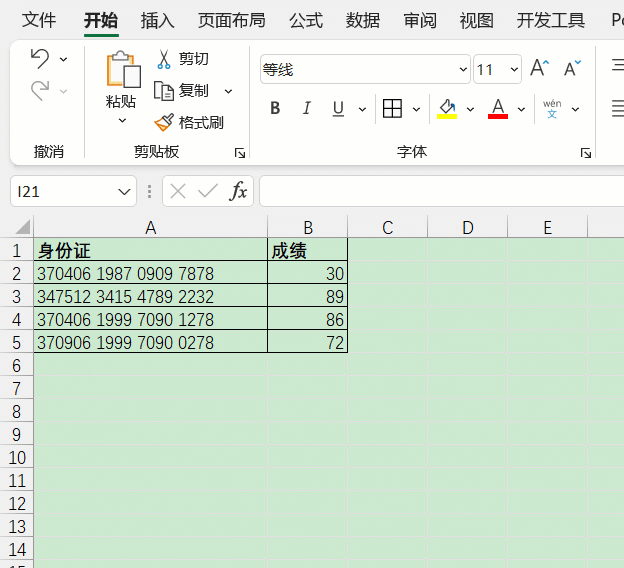## 3、去除首尾空格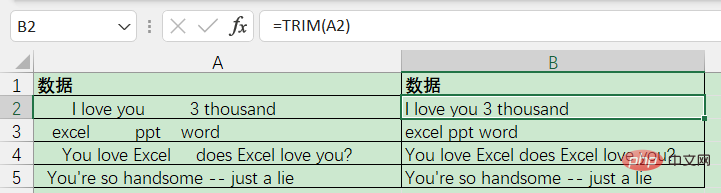B2单元格输入以下公式即可：

`=TRIM(A2)`

## 4、删除系统导出的空格

`=UNICHAR(ROW(A1))`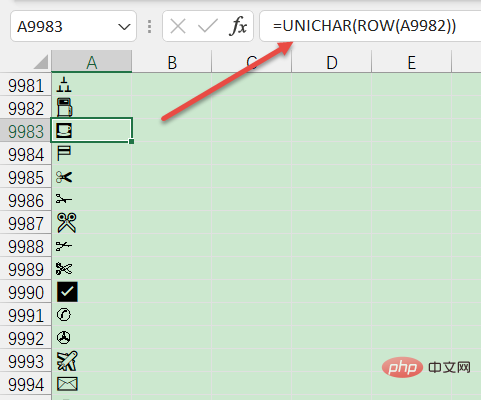`=CLEAN(TRIM(A1))`

CLEAN，在英语中是清洁打扫的意思，它可以清理掉一部分不可见的空格。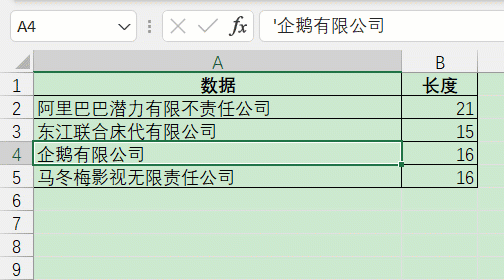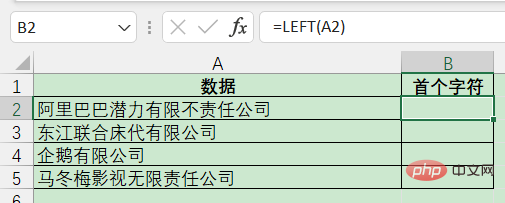`=SUBSTITUTE(A2,LEFT(\$A\$2),””)`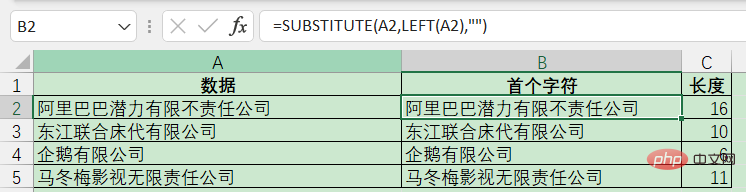`=SUBSTITUTE(A2,RIGHT(\$A\$2),””)`

`=SUBSTITUTE(SUBSTITUTE(A2,RIGHT(\$A\$2),””),LEFT(\$A\$2),””)`﻿